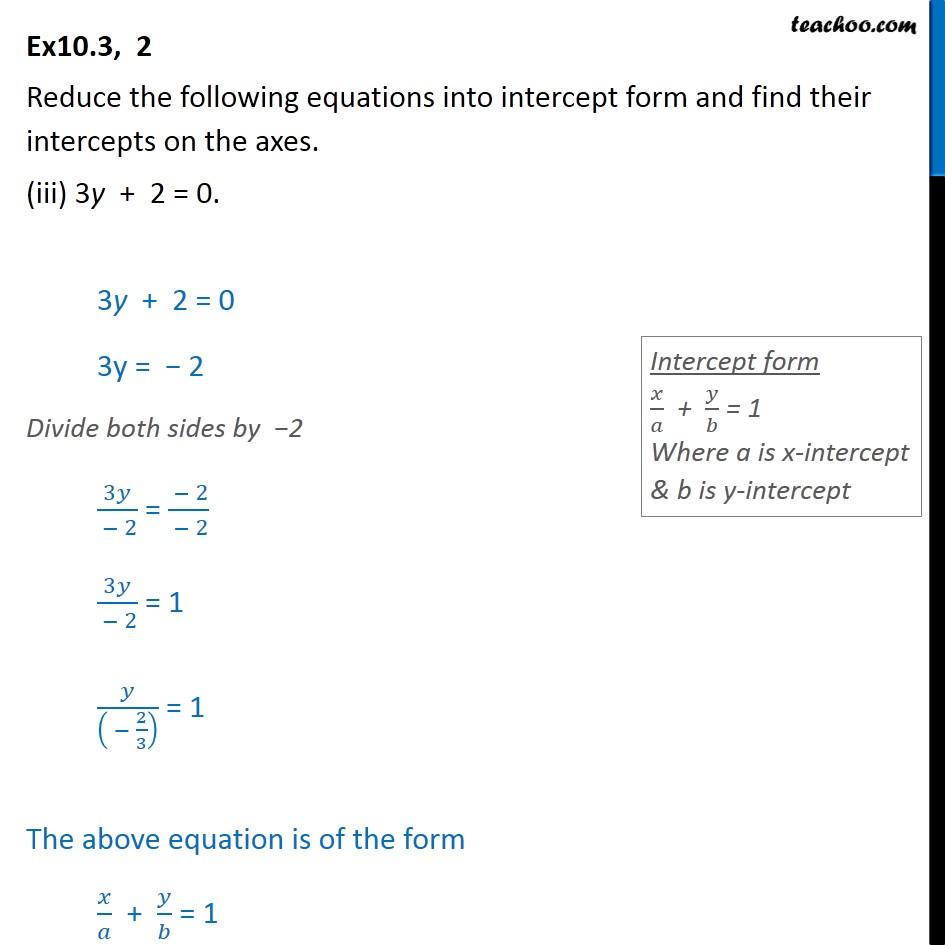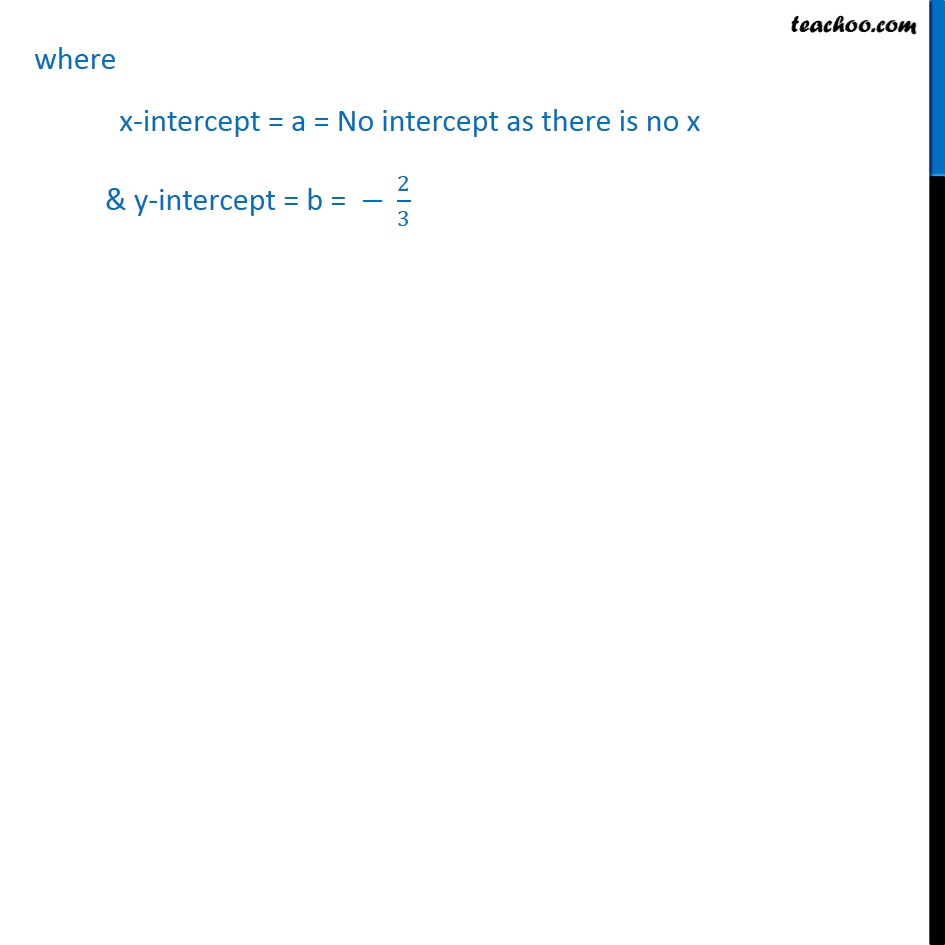Ex 10.3

Chapter 10 Class 11 Straight Lines
Serial order wiseMaths Crash Course - Live lectures + all videos + Real time Doubt solving!

### Transcript

Ex10.3, 2 Reduce the following equations into intercept form and find their intercepts on the axes. (iii) 3y + 2 = 0. 3y + 2 = 0 3y = 2 Divide both sides by 2 3 /( 2) = ( 2)/( 2) 3 /( 2) = 1 /(( 2/3) ) = 1 The above equation is of the form / + / = 1 where x-intercept = a = No intercept as there is no x & y-intercept = b = 2/3